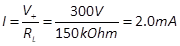A very useful information, generally provided in the datasheet of an electronic vacuum tube, is the average anode characteristics graph. Figure 7 shows this graph for a 12AX7 vacuum tube. In the graph, each black plot is obtained by maintaining fixed the grid voltage Vg, and varying the anode voltage Va. Each black plot is respectively tagged with the corresponding grid voltage Vg used. The graph shows, for a fixed grid voltage Vg, the relationships between the anode voltage Va and the anode current Ia. When Va increases, Ia increases as well along the plot corresponding to the chosen Vg.

In a voltage amplifier, as the one in Figure 6, once the power supply V+ is fixed, when the grid voltage Vg varies, the vacuum tube resistance varies and Va and Ia vary as well, as discussed before. The anode voltage Va and the current Ia vary together along a straight line, as for instance the green line in Figure 7. This line is called the loadline and is very useful for setting up the vacuum tube operating point, choosing the power supply voltage, and the load of the amplifier. The slope of the loadline depends on the load resistance RL. Its intersection, with the anode voltage axis, depends on the power supply V+.

The loadline, in case of a resistive load, can be drawn by connecting together the point corresponding to maximum anode voltage and minimum conduction, with the point corresponding to minimum anode voltage and maximum conduction, as discussed in the following example.

 Example 1: Determining the loadline Let us suppose the power supply is V+=300V. As an example, let us first consider the (non realistic) case where no load is applied to the anode, that is when RL=0. In this case, the anode voltage is always equal to the power supply voltage Va=V+. When Vg=-4.0, the corresponding plot in the graph indicates that at Va=300V there is no conduction, since Ia=0. If we vary the grid voltage from -4.0V to -1.5V, given that the anode voltage is always Va=300V, the current varies from 0 up to 3.15 mA, following the red vertical line, drawn in the figure. In fact, the plot corresponding to Vg=-1.5V indicates that when Va=300V, we have Ia=3.15 mA. Suppose now that the load applied between the anode and power supply is RL=150K Ohm. Let us first determine the point of minimum conduction. When the vacuum tube does not conduce, the tube internal resistance is infinite Rv=∞. Therefore, the voltage seen at the anode is Va=300V and current is zero. Let us now suppose that the vacuum tube does not offer any resistance. In this case, the anode voltage Va is zero  and the voltage drops from 300V to 0 through the load. The anode current is obtained using the Ohm law as. Accordingly, the loadline intersects the horizontal axis at 300V and the vertical axis at 2.0 mA, obtaining the green line in Figure 7. Grid voltage variations between 0V and -4V shift anode voltage and current along this line.

To experiment with loadlines of various vacuum tubes you can use my loadline calculator.

A load with a small impedance produces a more vertical loadline. Large load impedance makes a more horizontal loadline. Small impedance produces more current and less voltage swing, while large impedance mainly produces voltage variations.

Resuming, the loadline indicates what happens at the anode of a vacuum tube used as a voltage amplifier, when a negative voltage is applied to its grid. A signal applied to the grid is seen as a movement along the loadline.Figure 7: Average anode characteristic graph and loadline.Every black plot corresponds to the relationship between anode voltage (Va) and current from anode to cathode (Ia), at a fixed grid voltage. If the anode voltage is maintained fixed and the grid voltage varies, the current varies as well following a vertical line (for example along the red line). If a load is applied between the anode and the power supply, this forms a voltage divider with the vacuum tube and both voltage and current vary when the grid voltage varies (for example along the green line). The line along which the vacuum tube operates depends on the impedance of the load and is called the loadline.